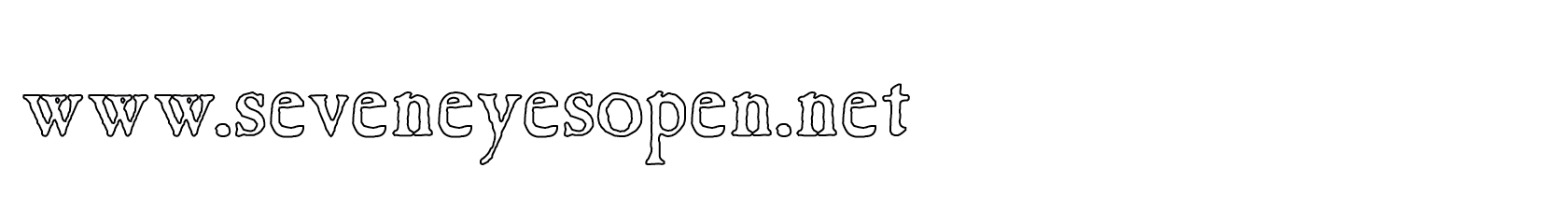None:
Polyps:
Strongs:MathematicsGod Does Play Dice Now the number of ways I may arrange the faces of a die (a cube) so as to generate distinct dice is 30. This is not too difficult to find, as I may place an arbitrary side of a die facing me, then place any one of the remaining 5 numbers on the opposite face. It makes no difference which number would go on the “top” of the die, this is also arbitrary (I may rotate the die freely.) Then to finish, I note I have 6 = 3x2x1 permutations for the last three numbers on the last three faces. Then, the number of distinct dice is 30 = 5x3x2x1 Now, how many octal groups are there in seven symbols? I will simply use my familiar triples used throughout the book, and choose (count the arrangements of) the elements of a G-set upon which the octal acts. Those G-set members may be arbitrarily assigned up to the number of arrangements of a generalised octal in seven symbols. I.e. The octal acts as a permutation representation of the G-set's members So, does God play dice?. I may clearly choose the first two symbols as members of my first K4 group, and freely so, as there must be a product between any two elements. (There must be one K4 subgroup containing them. A K4 subgroup is isomorphic to the ordered pair C2 x C2.) So given my first two symbols from seven a, b say, are arbitrary. I may choose five (remember, five) elements for “c” to make my first K4 subgroup. Then once again, I may arbitrarily pair any two of the remaining four symbols (say d and e) to any one of a, b or c in that first group of three; (giving 3*5 = 15) the result is that I now have the K4 subgroup [a,d,e] say, which fixes without question a third K4 subgroup [a,f,g]. (Every two K4 subgroups share only one common element. Each element is in three K4 subgroups,) Then in any one of the remaining two elements of my first group (as b, c above) I may choose an arbitrary element of one from the other two of those first three subgroups (say d or e, as every product is in a K4 subgroup and these were not so counted yet); and fix the last element from the remaining subgroup (say the two choices of f or g). This gives me one pair of {[b,d,f], [b,d,g]} or {[b,e,f], [b,e,g]}. This last subgroup uniquely fixes the rest of the seven of that octal in closure. Likewise I count them 5*3*2*1 = 30 “arrangements” or octal groups in seven symbols. Of course, God does not gamble but I find it helpful to remember the number of octals this way! It is worthy to note that there are only two legal dice; I may choose any number for the face toward me, but then the face opposite must total 7 with that first number. The next number is arbitrary and the face opposite that is also uniquely determined; leaving only two possibilities remaining. Each die is then either "left handed" or "right handed". There are also two complement left and right handed octals for every choice of multiplication, (each a group C7 that preserves the octal's subgroups. Though there are eight such possible multiplications over each octal.) So, God is either "left or right handed". I am not a gambling man, but I like that. Continue To Next Page Return To Section Start Return To Previous Page'